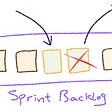# Quantum Coin Toss

`import chancesimport random# use pseudo randomness to pick either even or oddrandom.choice(['even', 'odd'])# use pseudo randomness to pick either head or tailrandom.choice(['heads', 'tails'])# set the `max` and `n` values for the random generatorrand = chances.Randomizer(1000000, 1)# use quantum mechanical randomness to pick a numberrand.quantum()`

In the first two lines of the actual code, we decide if even or odd will be head or tails.

In the third line, we initiate the random number generator to give a single value that is not greater than 1,000,000.

In the fourth line, we do the actual pick from a real-time stream of quantum mechanically appearing numbers.

Putting it all together, if we get as output`even` from the first line, and `heads` from the second line, and `20301` from the fourth line, we then know we have `tails` .

To express the some properly in a function that directly answers the question between two arbitrary alternatives:

`def quantum_coin_toss(a, b):        '''Quantum coin tosser    a | str or int or list or dict | any value or set of values    b | str or int or list or dict | any value or set of values        '''        import chances    import random        # use pseudo randomness to pick either even or odd    even_or_odd = random.choice(['even', 'odd'])        # use pseudo randomness to pick either head or tail    a_or_b = random.choice([a, b])        # set the `max` and `n` values for the random generator    rand = chances.Randomizer(1000000, 1)        # use quantum mechanical randomness to pick a number    quantum_random_number = rand.quantum()        # handle the case where quantum random number is even    if quantum_random_number % 2 == 0:                if even_or_odd == 'even' and a_or_b == a:            return a                elif even_or_odd == 'even' and a_or_b == b:            return b                elif even_or_odd == 'odd' and a_or_b == a:            return b                elif even_or_odd == 'odd' and a_or_b == b:            return a            if quantum_random_number % 2 != 0:                if even_or_odd == 'even' and a_or_b == a:            return b                elif even_or_odd == 'even' and a_or_b == b:            return a                elif even_or_odd == 'odd' and a_or_b == a:            return a                elif even_or_odd == 'odd' and a_or_b == b:            return b`

--

--

--

## More from Mikko

Worked with machine intelligence for 15 years, and built the interwebs for 25. Nothing here is my own.

Love podcasts or audiobooks? Learn on the go with our new app.

## Redis Fragmentation and Optimization## Elegant Python code reproduction of "most common words from a story"## Run your own high performance EBS wherever Kubernetes runs## The Passion for Developer Advocacy in the Kin Ecosystem## Modifying sprint backlog during the planned sprint## Architecture and Workflow Overview## Mikko

Worked with machine intelligence for 15 years, and built the interwebs for 25. Nothing here is my own.

## Artificial Moon## Quantum Error Correction and the Black Hole Information Paradox## NBA Big Board 2.0## From Mars to Earth